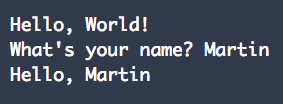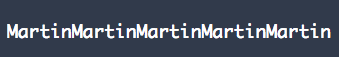# How to Store Data in Python

## A tutorial on storing data in Python.In this lesson we're going to look at how to store data. If you haven't read the previous lessons yet, you can find them here:

Lesson 1: Hello Python

Python Lesson 2: Control Flow and Repetition (Loops)

To store data we use something called a variable. Variables can store all different types of information, from information entered by the user to scores in a game. They can also be updated to keep track of changes.

For example, in a game, a variable can store the number of attempts or lives a player has left to complete the game. The most common data types used in Python are numbers and strings.

## Creating variables

In some programming languages you have to declare a variable before using them or define the information that will be stored in it, e.g., a number.

However, in Python we just need to type the name of our variable, followed by an equals sign and a value to assign to it.

For example:

``````age = 31
``````

This is called assigning a value to a variable. Python knows the type of the value assigned to a variable, so will automatically know how to handle the assigned value. It will throw an error if you try and do anything silly with it, like trying to divide your name by your age. The type of value a variable holds doesn't even have to remain the same throughout a program. It can be changed by another assignment like above.

## Tips for naming variables

Variables can be named anything you want. However, to make it more readable both for yourself and for others reading it, it's best to use meaningful variables names.

For example, in my previous example I used:

``````age = 31
``````

From the name it is obvious that it is storing someone's age (if it's been named meaningfully). However, what would happen if it was called x? Would you be able to easily guess what the variable was being used for? Imagine a whole program written using variables a, b, c, …, etc. It would be difficult for anyone to understand it, that hadn't written it. It might also be hard to understand for the person that did write it, as it may have been a long time since they've looked at it. Variable names can contain letters, numbers and underscores but they can't begin with a number. Here are some guidelines to follow when naming variables:

• Start variable names with a letter (they can also begin with underscores but this represents a special type of variable I'll discuss in a much more advanced blog)

• The rest of the name can be made up of a combination of letters, numbers and underscores

• Spaces can't be used, so use underscores (_) instead

• Python is case sensitive so age and Age will be treated as different variables

• The style guide for Python, PEP 8, states that variables should be all lowercase with underscores separating the words to improve readability

• Avoid words Python uses as commands, such as print. In some cases you might want to do this but this is for much more advanced cases. Again, I will discuss this in a much more advanced blog.

## Numbers

There are several different number types in Python. The most commonly used ones are integer and float. There's also long and complex, which will be covered in a later blog. Integers are whole numbers, e.g., 1, whereas floats, have a decimal point, e.g., 1.5.

Variables that have numbers assigned to them, can be used in calculations, so Python can be used just like a calculator. Python supports various arithmetic operators. The main ones being addition, subtraction, multiplication and division. The symbols for addition and subtraction will be the same as what you're used to, + and -, respectively. However, multiplication and division are represented using * and /, respectively.

Try entering some basic calculations into IDLE. If you use a variable name in your code, anywhere that isn't an assignment, it will be evaluated as the value assigned to it.

For example:

``````x = 10
y = x * 5
``````

Will be evaluated as:

``````x = 10
y = 10 * 5
``````

To see the result we can use print.

``````print(y)
``````

## Strings

A string is any piece of data that is made up of a sequence of letters or other characters. For example, words and sentences. Strings can even include numbers. In Python any data between quotation marks, double (“”) or single (‘'), is treated as a string. To assign a string to a variable, you use the same syntax as for integers but put the data in quotation marks. For example,

``````name = "Martin"
``````

Note how I've used a meaningful variable name so my code is easily readable. I know the value assigned to this variable must be someone's name. In this case, my name. Try assigning some strings to variables and printing them. For example,

``````message = 'Hello, World!'
print(message)
``````

We can also use + symbol to add strings together. Remember our first program?

``````person = input("What's your name? ")
print("Hello, " + person)
``````

Which outputs:We can also use the multiply operator with strings.

For example:

``````name = "Martin"
print(name * 5)
``````

Which outputs:Let's add to our program from my first blog to create a simple chat bot. Copy or type the following code:

The first line asks the user their name and assigns what they type to the person variable. The other input lines work similarly. Try adding some more questions of your own.

In my next blog, I'll be talking about conditional statements. With these we can update our very basic chat bot to give smarter responses to questions by giving an answer based on what the user has said.